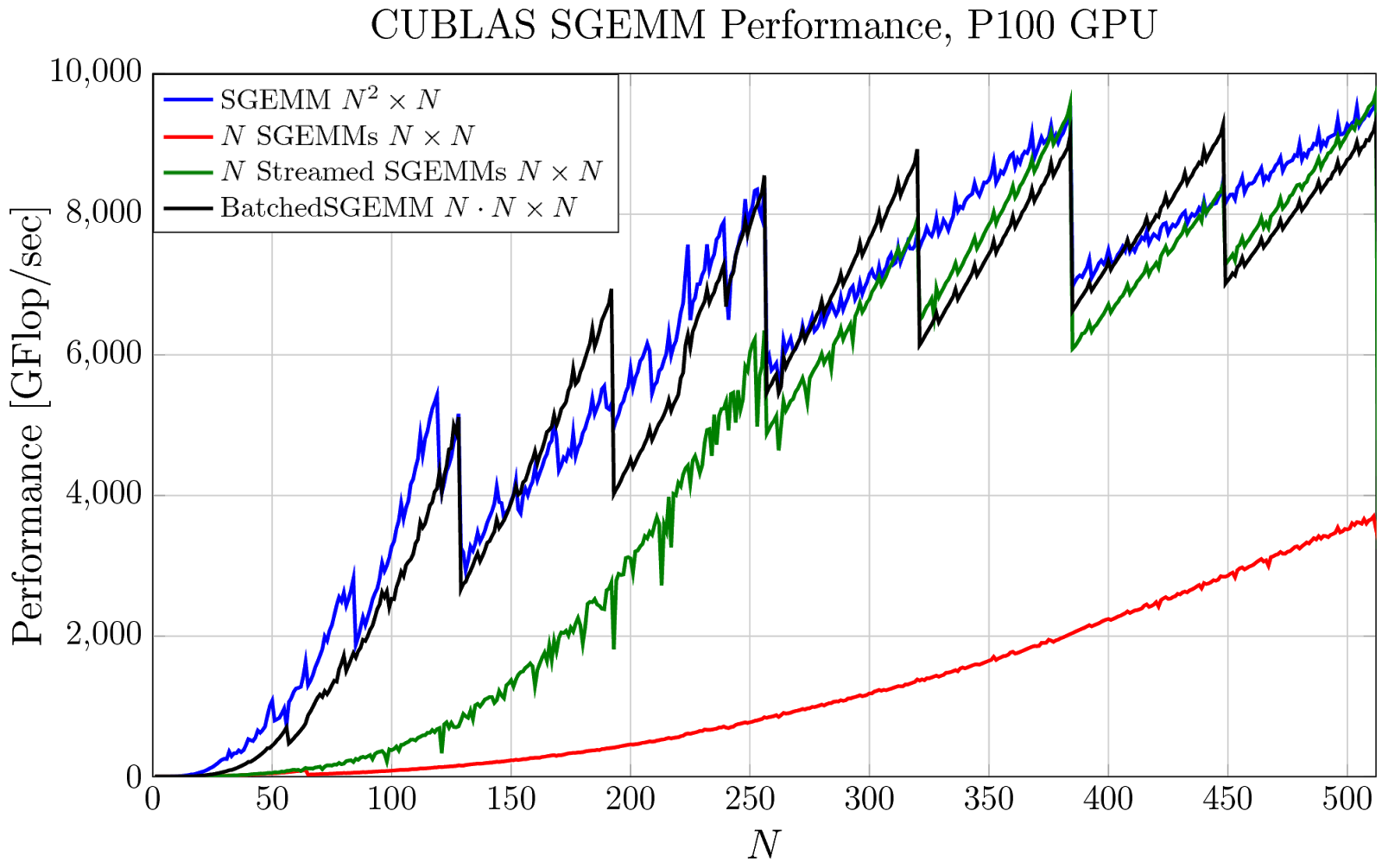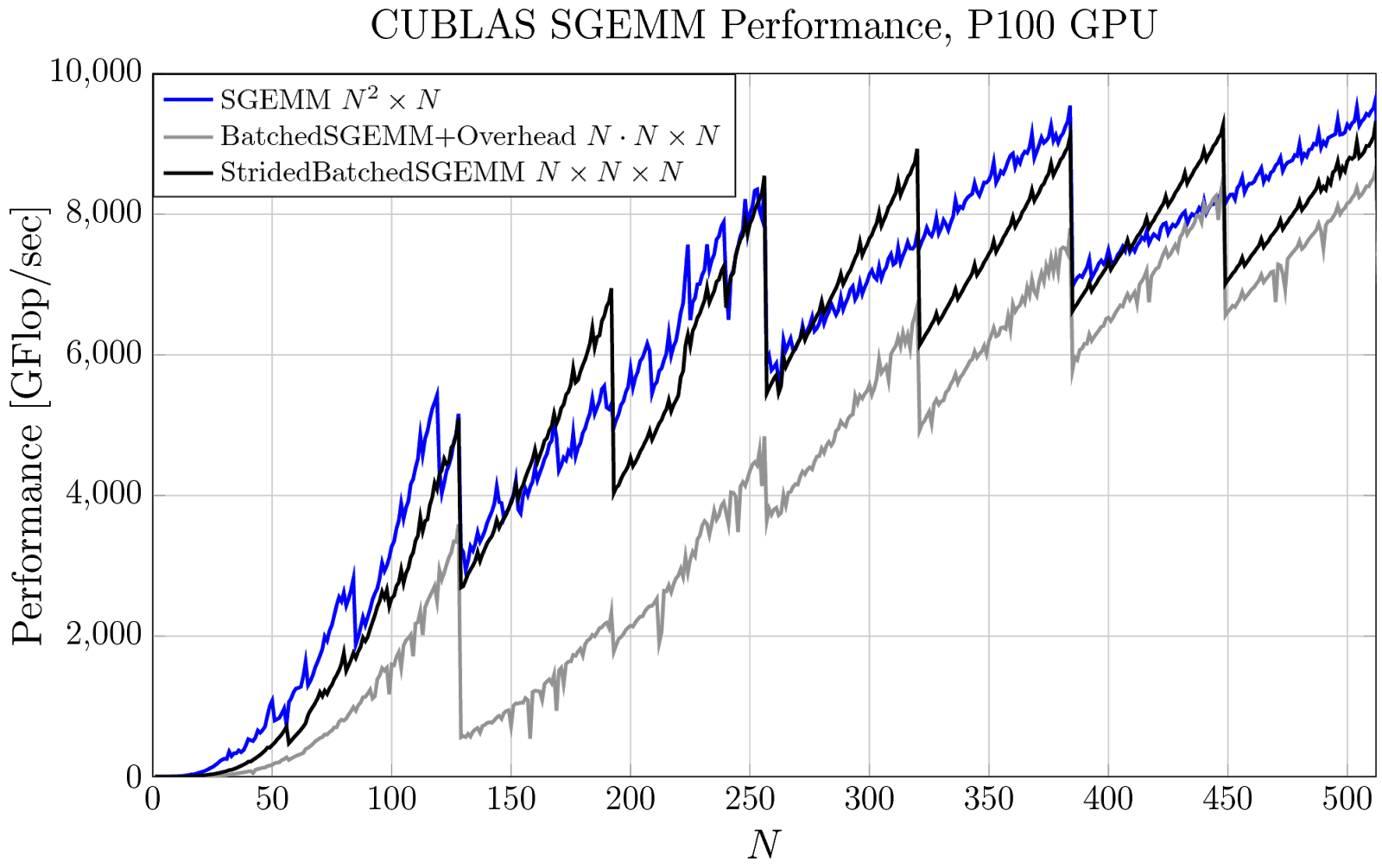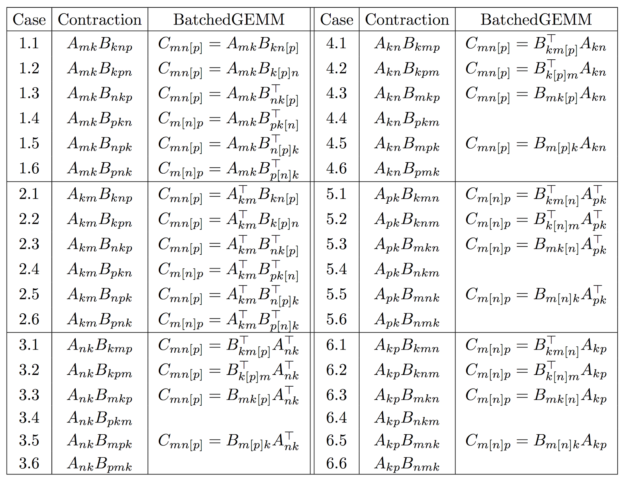# Pro Tip: cuBLAS Strided Batched Matrix MultiplyThere’s a new computational workhorse in town. For decades, general matrix-matrix multiply—known as GEMM in Basic Linear Algebra Subroutines (BLAS) libraries—has been a standard benchmark for computational performance. GEMM is possibly the most optimized and widely used routine in scientific computing. Expert implementations are available for every architecture and quickly achieve the peak performance of the machine. Recently, however, the performance of computing many small GEMMs has been a concern on some architectures. In this post, I detail solutions now available in cuBLAS 8.0 for batched matrix multiply and show how it can be applied to efficient tensor contractions, an interesting application that users can now be confident will execute out-of-the-box with the full performance of a GPU.

## Batched GEMM

The ability to compute many (typically small) matrix-matrix multiplies at once, known as batched matrix multiply, is currently supported by both MKL’s cblas_<T>gemm_batch and cuBLAS’s cublas<T>gemmBatched. (<T> in this context represents a type identifier, such as S for single precision, or D for double precision.)

Both of these interfaces support operations of the form$C[p] = \alpha \, \text{op}(A[p]) \, \text{op}(B[p]) + \beta \, C[p],$

where A[p], B[p], and C[p] are matrices and op represents a Transpose, Conjugate Transpose, or No Transpose. In cuBLAS, the interface is:

<T>gemmBatched(cublasHandle_t handle,
cublasOperation_t transA, cublasOperation_t transB,
int M, int N, int K,
const T* alpha,
const T** A, int ldA,
const T** B, int ldB,
const T* beta,
T** C, int ldC,
int batchCount)

For reference, <T>gemmBatched implements the following computation (along with all of the variants associated with the transpose arguments).

for (int p = 0; p < batchCount; ++p) {
for (int m = 0; m < M; ++m) {
for (int n = 0; n < N; ++n) {
T c_mnp = 0;
for (int k = 0; k < K, ++k)
c_mnp += A[p][m + k*ldA] * B[p][k + n*ldb];
C[p][m + n*ldc] = (*alpha)*c_mnp + (*beta)*C[p][m + n*ldc];
}
}
}

This is extremely versatile and finds applications in LAPACK, tensor computations, machine learning (RNNs, CNNs, etc), and more. Both the MKL and cuBLAS implementations are optimized for small matrix sizes as well.Figure 1: Performance of four strategies for computing N matrix-matrix multiplications of size NxN.

In Figure 1, I’ve plotted the achieved performance on an NVIDIA Tesla P100 GPU of four evaluation strategies that use some form of cuBLAS SGEMM. The blue line shows the performance of a single large SGEMM. But, if many smaller SGEMMs are needed instead, you might simply launch each smaller SGEMM separately, one after another. This is plotted in red and the achieved performance is quite poor: there are many kernels launched in sequence and the small matrix size prevents the GPU from being fully utilized. This can be improved significantly by using CUDA streams to overlap some or all of the kernels—this is plotted in green—but it is still very costly when the matrices are small. The same computation can be performed as a batched matrix multiply with a single call to cublasSgemmBatched, plotted in black, where parity with the original large SGEMM is achieved!

One issue with the pointer-to-pointer interface, in which the user must provide a pointer to an array of pointers to matrix data, is the construction and computation of this data structure. Code, memory, and time has to be invested to precompute the array of pointers. In a common case, we end up with something like the following code.

T* A_array[batchCount];
T* B_array[batchCount];
T* C_array[batchCount];
for (int p = 0; p < batchCount; ++p) {
A_array[p] = A + p*strideA;
B_array[p] = B + p*strideB;
C_array[p] = C + p*strideC;
}

Which clutters code and costs performance, especially when the pointers can’t be precomputed and reused many times. Even worse, in cuBLAS the matrix pointers must exist on the GPU and point into GPU memory. This means that the above precomputation translates into (1) GPU memory allocation, (2) Pointer offset computations, (3) GPU memory transfers/writes, and (4) GPU memory deallocation. Many of these steps are expensive, optional, could imply synchronization, and are generally frustrating.

Fortunately, as of cuBLAS 8.0, there is a new powerful solution.

## Strided Batched GEMM

For the common case shown above—a constant stride between matrices—cuBLAS 8.0 now provides cublas<T>gemmStridedBatched, which avoids the auxiliary steps above. The interface is:

<T>gemmStridedBatched(cublasHandle_t handle,
cublasOperation_t transA, cublasOperation_t transB,
int M, int N, int K,
const T* alpha,
const T* A, int ldA, int strideA,
const T* B, int ldB, int strideB,
const T* beta,
T* C, int ldC, int strideC,
int batchCount)

For reference, <T>gemmStridedBatched implements the following computation (along with all of the variants associated with the transpose arguments).

for (int p = 0; p < batchCount; ++p) {
for (int m = 0; m < M; ++m) {
for (int n = 0; n < N; ++n) {
T c_mnp = 0;
for (int k = 0; k < K, ++k)
c_mnp += A[m + k*ldA + p*strideA] * B[k + n*ldB + p*strideB];
C[m + n*ldC + p*strideC] =
(*alpha)*c_mnp + (*beta)*C[m + n*ldC + p*strideC];
}
}
}

The interface is slightly less versatile, but the performance packs the same punch as cublas<T>gemmBatched, while avoiding any overhead from precomputation.Figure 2: Performance of three strategies for computing N matrix-matrix multiplications of size NxN.

Figure 2 plots the achieved performance of three strategies that use some form of cuBLAS SGEMM. In blue, I’ve again plotted the performance of a single large SGEMM. In gray, the performance of cublasSgemmBatched, but this time I’ve included the overhead—the time it takes to allocate, compute, and transfer the pointer-to-pointer data structure to the GPU. The overall performance is greatly impacted, especially when we have only a few small matrices. In many cases, the same computation can be performed with a single call to cublasSgemmStridedBatched, drawn in black, without any required precomputation and the original batched GEMM performance is achieved again!

It’s interesting to note that the interface of cublas<T>gemmStridedBatched allows only a strict subset of the operations available in the pointer-to-pointer interface of cublas<T>gemmBatched, but offers a number of benefits:

• You don’t have to precompute pointer offsets and/or allocate/deallocate auxiliary memory to use the interface. This is especially beneficial in GPU computing where allocation and transfer can be relatively more expensive and could cause undesired synchronization.
• Regular blocked matrices such as block-diagonal matrices, block-tridiagonal, or block-Toeplitz can be applied straightforwardly. Moreover, operations of this form appear frequently in tensor computations [Shi 2016], physics computations such as FEM and BEM [Abdelfattah 2016], many numerical linear algebra computations [Dong 2014, Haidar 2015], and machine learning.
• The door is opened for even more optimizations in our implementation. For example, if a matrix stride is zero, then the batched GEMM is multiplying many matrices by a single matrix. In principle, the single matrix could be read once and reused many times. Other clever reorderings of how the computation is actually performed are now available to implementers.

## Application: Tensor Contractions

A very simple application of this new primitive is the efficient evaluation of a wide range of tensor contractions. Table 1 lists all possible single-index contractions between an order-2 tensor (a matrix) and an order-3 tensor to produce an order-3 tensor.Table 1: All possible single-index contractions between an order-2 tensor (a matrix) and an order-3 tensor to produce an order-3 tensor.

Elements are stored in “generalized column-major” order:$C_{mnp} \equiv C[m + n \cdot \text{ldC1} + p \cdot \text{ldC2}].$

A single call to GEMM can perform 8 out of the 36 contractions, but only if the data storage is compact! On the other hand, a single call to stridedBatchedGEMM can perform 28 out of the 36 cases, even when the storage is non-compact! In the table, the batched index is written in brackets [.] and the transposition arguments are written over their “batched matrix”.

For example, to compute Case 6.1, you can write the following.

cublasSgemmStridedBatched(cublas_handle,
CUBLAS_OP_T, CUBLAS_OP_N,
M, P, K,
1.f,
B, ldB1, ldB2,
A, ldA, 0,
0.f,
C, ldC2, ldC1,
N);

This computes the following, which is exactly what we wanted!

for (int n = 0; n < N; ++n) {
for (int m = 0; m < M; ++m) {
for (int p = 0; p < P; ++p) {
T c_mnp = 0;
for (int k = 0; k < K, ++k)
c_mnp += B[k + m*ldB1 + n*ldB2] * A[k + p*ldA];
C[m + n*ldC1 + p*ldC2] = c_mnp;
}
}
}

Notice the clever use of a matrix stride of zero, applying a transpose to each B-matrix in the batch, flipping the input arguments A and B in order to get the right expression, and swapping the ldC1 and ldC2 parameters to get a banded output rather than a blocked output from each GEMM. For more information see [Shi 2016], which details the need for this primitive, more performance profiles, and additional applications such as in unsupervised machine learning.

Calling cublas<T>gemmStridedBatched avoids having to manually reshape (e.g. using copy or geam) the tensors into matrices in order to use GEMM, saves an enormous amount of time (especially for small tensors), and executes just as fast as GEMM does! This is beautiful.

## Getting Started with Batched Matrix Multiply

Batched and strided batched matrix multiply (GEMM) functions are now available in cuBLAS 8.0 and perform best on the latest NVIDIA Tesla P100 GPUs. You can find documentation on the batched GEMM methods in the cuBLAS Documentation to get started at peak performance right away!

For more information about the motivation, performance profiles, and applications of batched GEMMs in machine learning and tensor computations, see Yang Shi’s HiPC 2016 paper. To hear about why these ideas are critically important in my ongoing work with tensors and structured dense matrix factorizations, see my upcoming talk at GTC 2017, Low-Communication FFT with Fast Multipole Method”.

### References

[Shi 2016] Y. Shi, U. N. Niranjan, A. Anandkumar, and C. Cecka. Tensor Contractions with Extended BLAS Kernels on CPU and GPU. In 2016 IEEE 23rd International Conference on High Performance Computing (HiPC), pages 193–202, Dec 2016. ieee.org/document/7839684/

[Abdelfattah 2016] Abdelfattah, A., Baboulin, M., Dobrev, V., Dongarra, J., Earl, C., Falcou, J., Haidar, A., Karlin, I., Kolev, T., Masliah, I., Tomov, S.: High-performance tensor contractions for GPUs. In: International Conference on Computational Science (ICCS 2016). Elsevier, Procedia Computer Science, San Diego, CA, USA, June 2016 hgpu.org/?p=15361

[Haidar 2015] A. Haidar, T. Dong, S. Tomov, P. Luszczek, and J. Dongarra. A Framework for Batched and GPU-resident Factorization Algorithms Applied to Block Householder Transformations International Supercomputing Conference IEEE-ISC 2015, Frankfurt, Germany.

[Dong 2014] T. Dong, A. Haidar, S. Tomov, and J. Dongarra. A Fast Batched Cholesky Factorization on a GPU ICPP 2014, The 43rd International Conference on Parallel Processing 2014.

[Relton 2016] Samuel D Relton, Pedro Valero-Lara, and Mawussi Zounon. “A Comparison of Potential Interfaces for Batched BLAS Computations,” hgpu.org/?p=16401